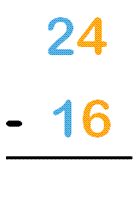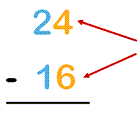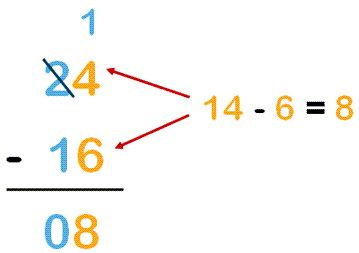# Two-digit Subtraction with Regrouping

## What is regrouping?

To regroup means to re-organize something into a new group or groups. Applied to subtraction, this means to regroup sums of numbers into a new group or groups. In subtraction, we sometimes call regrouping borrowing, as you borrow from the number to the left. This will all make sense when you see the video example below.

Regrouping in subtraction is applied when you subtract numbers in columns, so that the ones are stacked above each other, the tens are stacked above each other, the hundreds are stacked above each other, etc.

We now think of the ones as one group, the tens as one group, the hundreds as one group, etc.

## Regrouping in subtraction

Regrouping, or borrowing, in subtraction is best explained with an example.

Let’s show you how with this example: 24 – 16

Now, let’s put them in columns:Look at the ones – we’re trying to take 6 away from 4.That’s not possible, so we have to borrow 10 from the tens column. Now we can now take 6 away from 14.

Now let’s subtract the numbers in the tens column: one 10 minus one 10, which is zero.The answer for 24 – 16 = 8.

## 2-digit subtraction with regrouping video

We’ve created a 60 second video to demonstrate visually how to subtract two 2-digit numbers with borrowing:

## Grade 2 worksheets to practice 2-digit subtraction with borrowing

We’ve created a series of worksheets for students to practice regrouping when two 2-digit numbers.Become a Member

This content is available to members only.